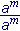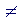Lesson 20  Section 2

Back to Section 1

Exponent 0

Scientific notation

Exponent 0

Any number (except 0) with exponent 0 is defined to mean 1.

a0 = 1

Problem 8.   Evaluate the following..

Do the problem yourself first!

 a) 50  =  1. b) (−5)0  =  1. c) −50  =  −1. d) (½)0  =  1.
 e) 3· 100  =  3· 1 = 3. f) (3· 10)0  =  1. g) −3· 100  =  −3· 1 = −3.

h)  5 + 50(5 + 50) =  5 + 1(5 + 1) = 5 + 6 = 11.

See the order of operations.

Why do we define a0 as 1?

For one thing, we have this rule:= am − m = a0. But (when a0),= 1.

Therefore, we must define a0 as 1.

As for 00, that could be any number! For,= Any number. Lesson 6

But= 0

Therefore we must conclude that 00 could be any number.

Problem 9.   Use the rules of exponents to evaluate the following.

 a)= 107 − 2 + 3 − 8 = 100 = 1
 b)= 22 − 8 + 4 + 3 − 6 + 5 = 20 = 1

Scientific notation

"Scientific notation" has one non-zero digit to the left of the decimal point.

2.345

That number is written in scientific notation.  There is one digit to the left of the decimal point -- 2 -- and it is not 0.

In general, a number written in scientific notation will be multiplied by 10 raised to an exponent.

Example 1.   6.45 × 103.  This is the scientific notation for what number?

Answer.   Since the exponent is positive, we will be multiplying 6.45.  To multiply a decimal by a power of 10, move the decimal point right the number of places indicated by the exponent:

6.45 × 103 = 6,450

Move the point three places right.  To do that, we must add on a 0.(Skill in Arithmetic, Lesson 4.)

Example 2.   6.45 × 10−3.  This is the scientific notation for what number?

Answer.   Since the exponent is negative, we will be dividing 6.45.  (For,
 10−3 = 1   1000 .)  To divide a decimal by a power of 10, move the decimal

point left the number of places indicated by the exponent:

6.45 × 10−3 = .00645

Move the point three places left.  To do that, we must add on 0's.

Problem 10.   Each of these is written in scientific notation.  Multiply out.

 a) 2.401 × 103  =  2,401 b) 1.006 × 102  =  100.6 c) 7.01 × 105  =  701,000 d) 4.986 × 10  =  49.86 e) 3.85 × 10−4  =  .000385 f) 1.8 × 10−1  =  .18 g) 6.0 × 10−3  =  .006 h) 4.3 × 10−8  =  .000000043

Example 3.   Write in scientific notation.

a)   562.4 = 5.624 × 102

To change  562.4  into  5.624, we moved the decimal point two places left.  To compensate, we must multiply by 10 with exponent +2.

b)   .00395 = 3.95 × 10−3

To change  .00395  into  3.95, we moved the decimal point three places right.  To compensate, we must multiply by 10 with exponent −3.

Problem 11.   Write in scientific notation.

 a) 1234 = 1.234 × 103 b) 12.34 = 1.234 × 10 c) 219,000,000 = 2.19 × 108 d) 0.000081 = 8.1 × 10−5 e) 0.00395 = 3.95 × 10−3 f) 0.000000002 = 2.0 × 10−9Back to Section 1

Next Lesson:  Algebraic fractions

Please make a donation to keep TheMathPage online.
Even \$1 will help.